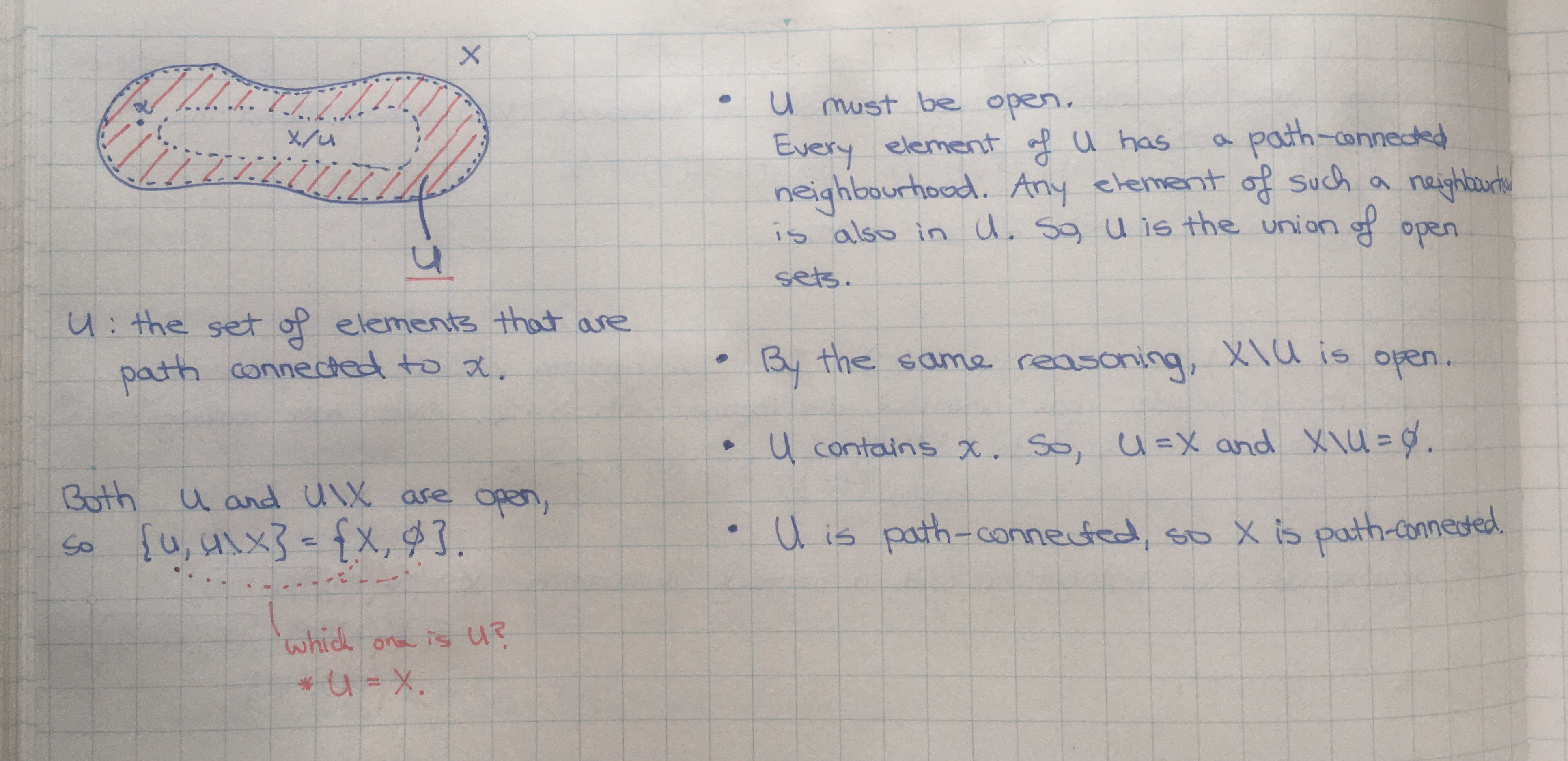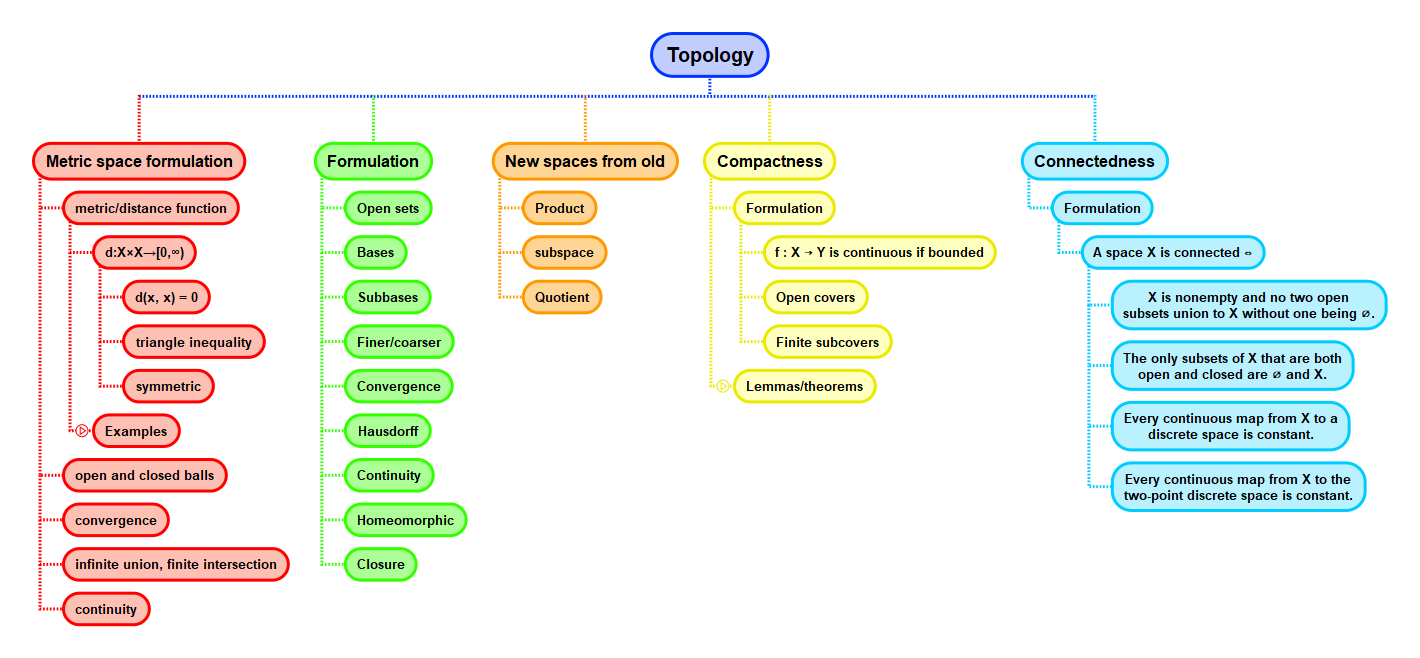Show Question
Math and science::Topology

# Path-connectedness. 4 statements

Path-connectedness implies connectedness, the converse is not generally true through.

The topologist's sine curve is an example of a space that is connected but not path-connected. Leinster covers the topologist's sine curve in some detail.

Below, we introduce an iff statement that does hold. It has the form: something ∧ connected ⟺ path-connected. After this, 3 conditions that each imply path-connectedness are presented.

#### 1. Path-connected ⟺ connected and all points have a path-connected neighbourhood

Let $$X$$ be a topological space. $$X$$ is path-connected if and only if $$X$$ is connected and every point of $$X$$ has at least one path-connected neighbourhood.

2. Corollary. Every connected open subset of $$\mathbb{R}^d$$ is path-connected.

#### 3. Path-connectedness and continuous functions

Let $$f : X \to Y$$ be a continuous map of topological spaces. If $$X$$ is path-connected, then so is $$fX$$.

#### 4. Path-connectedness and products

The product of two path-connected spaces is path-connected.

Proofs

#### Path-connected ⟺ connected and all points have a path-connected neighbourhood

Let $$X$$ be a topological space.

##### Forward implication

Assume that $$X$$ is path-connected. As shown in a previous card, $$X$$ is connected as it is path-connected. In addition, we have a path-connected neighbourhood for every point as the set $$X$$ is a neighbourhood for every point and $$X$$ is path-connected.

##### Reverse implication

Assume $$X$$ is connected and all points have a path-connected neighbourhood. Let $$x \in X$$ and let $$U$$ be the set of all elements having a path to $$x$$:

$U = \{ y \in X : \text{ there exists a path from } x \text{ to } y \text{ in } X \}$

Claim: both $$U$$ and $$X \setminus U$$ are open in $$X$$. Show this in two parts.

$$U$$ is open
Let $$y \in U$$. By assumption, there is an path-connected neighbourhood $$W$$ of $$y$$. Let $$w \in W$$. There is a path from $$x$$ to $$w$$ as we can join a path from $$x$$ to $$y$$ and a path from $$y$$ to $$w$$. Thus, $$W \subset U$$. As every element of $$U$$ has a neighbourhood contained in $$U$$, $$U$$ must be open (A2.9 in Leinster's notes).
$$X \setminus U$$ is open
Let $$y \in X \setminus U$$. By assumption, there is a path-connected neighbourhood $$W$$ of $$y$$. $$W \cap U = \emptyset$$, as if there was an element $$t \in W \cap U$$ we could find a path from $$x$$ to $$y$$ by joining the path from $$x$$ to $$t$$ and the path from $$t$$ to $$y$$; but such a path would contradict $$y \notin U$$. We can now state that $$W \in X \setminus U$$, as $$W \subseteq X$$ and $$W \cap U = \emptyset$$. As every element of $$X \setminus U$$ has a neighbourhood contained in $$X \setminus U$$, $$X \setminus U$$ must be open (again by A2.9).

So, we have both $$U$$ and $$X \setminus U$$ being open. However, $$X$$ is connected, so one of the sets must be empty and the other must equal $$X$$. $$x$$ itself is in $$U$$, so $$U = X$$. $$U$$ is path-connected by definition, so $$X$$ is path-connected.## ContextLeinster, p79